Do you like the article?
Share it with others -
Use new possibilities of MetaTrader 5

# Calculating mathematical expressions (Part 1). Recursive descent parsers

13 October 2020, 15:00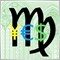0
4 872

When automating trading tasks, it can be required to provide the flexibility of computational algorithms at their execution stage. For example, when fine tuning programs distributed in a closed (compiled) mode, we can implement the selection of the objective function type from a wide range of possible combinations. In particular, this can be useful when optimizing an Expert Advisor or when quickly evaluating an indicator prototype. In addition to changing parameters in the dialog box, the users will also be able to change the calculation formula. In this case, we need to calculate the arithmetic expression from its textual representation, without changing the MQL program code.

This task can be solved through the use of various parsers allowing formula interpretation on the fly, their "compilation" into a syntax tree, generation of the so-called bytecode (sequence of computational instructions) and its further execution in order to calculate the result. In this article, we will consider several types of parsers and expression calculation methods.

### Formulation of the problem

Within this article, an arithmetic expression is a one-line sequence of data items and operators describing the relevant actions. Data items are numbers and named variables. Variable values can be set and edited from the outside, i.e. not in the expression but using special parser attributes. In other words, there is no assignment operator ('=') to store intermediate results. Below is the list of supported operators, shown in calculation priority order:

• !, - , + — unary logical negation, minus and plus
• () — grouping with parentheses
• *, /, % — multiplication, division and division modulo
• +, - — addition and subtraction
• >, <, >=, <= — more-less comparison
• ==, != — equal or not equal comparison
• &&, || — logical AND and OR (please note that priority is the same, so parentheses should be used)
• ?: — ternary conditional operator that allows you to branch calculations according to conditions

We will also allow the usage of MQL standard mathematical functions in expressions, which are 25 in total. One of them is the pow function for exponentiation. For this reason, the list of operators does not have the exponent operator ('^'). In addition, operator '^' only supports an integer power, while the function has no such restrictions. There is one more specific feature that distinguishes '^' from the other considered operators.

This is connected with the following. One of operator properties is associativity, which determines how operators of the same precedence are executed, either from the left or from the right. There are also other associativity types, but they will not be used in the context of the current task.

Here is an example of how associativity might affect the calculation result.

1 - 2 - 3

This expression does not explicitly indicate the order in which the two subtractions are performed. Since operator '-' is left-associative, first 2 is subtracted from 1 and then 3 is subtracted from the intermediate -1, which gives -4, i.e. this is equal to the following expressions:

((1 - 2) - 3)

If, hypothetically, operator '-' had right associativity, the operations would be performed in reverse order:

(1 - (2 - 3))

We would have 2. Fortunately, operator '-' is left-associative.

Thus, left or right associativity affects the expressions parsing and thereby complicates the algorithm. All the listed binary operators are left-associative, and only '^' is right-associative.

For example, the expression:

3 ^ 2 ^ 5

means that first 2 is raised to the power of 5, and then 3 is raised to the power of 32.

For simplicity, we will not use the exponent operator (and will use the pow function instead), so that algorithms will be implemented only taking into account left associativity. Unary operators are always right-associative and are therefore treated uniformly.

All numbers in our expressions (including those written as constants and as variables) will be of real type. Let us set the tolerance value for comparing them for equality. Numbers in logical expressions utilize a simple principle: zero is false, non-zero is true.

Bitwise operators are not provided. Arrays are not supported.

Here are some examples of expressions:

• "1 + 2 * 3" — calculating by operation priority
• "(1 + 2) * 3" — grouping using parentheses
• "(a + b) * c" — using variables
• "(a + b) * sqrt(1 / c)" — using function
• "a > 0 && a != b ? a : c" — calculating by logical conditions

Variables are identified by a name which is composed following the regular rules of MQL identifiers: they can consist of letters, numbers or underscores, and cannot start with a number. Variable names must not match built-in function names.

The input string will be analyzed character by character. General checks, such as whether a character belongs to letters or numbers, as well as error handling, variable setting and the extendable standard functions table, will be defined in the base class. All parser types will be inherited from this base class.

Let's consider all the parser classes. The articles present the classes with some simplifications. Full source codes are attached below.

### The parser base class (AbstractExpressionProcessor template)

This is a template class, since the expression analysis result can be not only a scalar value, but also a tree of nodes (objects of a special class), describing the expression syntax. We will consider later how this is done and what is the purpose of doing so.

First of all, the class object stores the expression (_expression), its length (_length), the current cursor position while reading the string (_index) and the current symbol (_token). It also has reserved variables indicating an error in the expression (_failed) and the value comparison precision (_precision).

```  template<typename T>
class AbstractExpressionProcessor
{
protected:
string _expression;
int _index;
int _length;
ushort _token;

bool _failed;
double _precision;

```

Special tables are provided for storing variables and links, however we will consider the relevant VariableTable and FunctionTable classes later.

```      VariableTable *_variableTable;
FunctionTable _functionTable;

```

The tables are pares with "key=value" pairs, where the key is the string with the variable or function name and the value is either a double (for variable) or a functor object (for tables).

The variable table is described by a reference because an expression cannot have variables. As for the functions table, the parser always has a minimum set of built-in functions (which a user can extend), that is why this table is represented by a ready-made object.

The table of standard functions is filled in the method:

```      virtual void registerFunctions();

```

The following section describes functions performing subtasks which are common to various parsers, such as switching to the next character, checking the character against the expected value (and showing an error if it does not match), sequentially reading digits that meet the format requirements, as well as some static auxiliary methods for classifying characters.

```      bool _nextToken();
void _match(ushort c, string message, string context = NULL);
virtual void error(string message, string context = NULL, const bool warning = false);

static bool isspace(ushort c);
static bool isalpha(ushort c);
static bool isalnum(ushort c);
static bool isdigit(ushort c);

```

All these functions are defined in this base class, in particular:

```  template<typename T>
bool AbstractExpressionProcessor::_nextToken()
{
_index++;
while(_index < _length && isspace(_expression[_index])) _index++;
if(_index < _length)
{
_token = _expression[_index];
return true;
}
else
{
_token = 0;
}
return false;
}

template<typename T>
void AbstractExpressionProcessor::_match(ushort c, string message, string context = NULL)
{
if(_token == c)
{
_nextToken();
}
else if(!_failed) // prevent chained errors
{
error(message, context);
}
}

template<typename T>
{
bool point = false;
while(isdigit(_token) || _token == '.')
{
if(_token == '.' && point)
{
error("Too many floating points", __FUNCTION__);
return false;
}
number += ShortToString(_token);
if(_token == '.') point = true;
_nextToken();
}
return StringLen(number) > 0;
}

```

Scientific notation with exponent is not supported in the parsing of numbers.

The class also declares the main 'evaluate' method which will be overridden in child classes. Here, it only initializes variables.

```    public:
virtual T evaluate(const string expression)
{
_expression = expression;
_length = StringLen(_expression);
_index = -1;
_failed = false;
return NULL;
}

```

This is the main class method, which receives a string with the expression as input and outputs the string processing result: a certain value if calculation was performed, or a syntax tree if analysis was performed.

The public interface of the class also contains constructors, to which it is possible to pass variables along with their values (as a string like "name1 = value1; name2 = value2; ..." or as a ready-made VariableTable object), methods for setting tolerance when comparing numbers, for getting the parsing success indication (showing that there was no syntax errors) and for accessing variables and functions tables.

```    public:
AbstractExpressionProcessor(const string vars = NULL);
AbstractExpressionProcessor(VariableTable &vt);

bool success() { return !_failed; };
void setPrecision(const double p) { _precision = p; };
double getPrecision(void) const { return _precision; };
virtual VariableTable *variableTable();
virtual FunctionTable *functionTable();
};

```

Please note that even if there are no syntax errors, an expression calculation can result in an error (for example, zero division, root of a negative value and so on). To control such situations, check if the result is a number using the MathIsValidNumber function. Our parsers must be able to generate appropriate value of different NaN (Not A Number) types, instead of crashing at runtime.

The easiest method is the recursive descent parser. So let us start with this parser.

### Recursive Descent Parser (ExpressionProcessor template)

A recursive descent parser is a set of mutually recursive functions which are called according to the rules describing separate operations. If we represent the syntax of some of the most common operations as a grammar in extended BNF notation (Extended Backus–Naur Form), then the expression can be represented as follows (each line is a separate rule):

```  Expr    -> Sum
Sum     -> Product { ('+' | '-') Product }
Product -> Value { ('*' | '/') Value }
Value   -> [0-9]+ | '(' Expr ')'

```

These rules are similar to the following in natural language. The expression parsing starts with the lowest-priority operation, which is addition/subtraction in this example. 'Sum' consists of two Product operands separated by the '+' or '-' signs, but the operation itself as well as the second operand are optional. 'Product' consists of two Value operands separated by '*' or '/'. Again, the operation and the second operand can be missing. 'Value' is any number consisting of digits or a nested expression specified in parentheses.

For example, the expression "10" (a number) will be expanded into the following sequence of rules:

```  Expr -> Sum -> Product -> Value -> 10

```

The expression "1 + 2 * 3" will have a more complex construction:

```  Expr -> Sum -> Product -> Value -> 1
|  '+'
-> Product -> Value -> 2
|  '*'
-> Value -> 3

```

According to the algorithm, grammar parsing is performed along with the matching between the input stream of characters and the operation rules. Parsing is performed from the main rule (the whole expression) to smaller components (down to individual numbers). The recursive descent parser belongs to the top-down class.

Our parser will support more operations (see the list in the first section). A separate method is reserved in the ExpressionProcessor derived class for each of the operations.

```  template<typename T>
class ExpressionProcessor: public AbstractExpressionProcessor<T>
{
public:
ExpressionProcessor(const string vars = NULL);
ExpressionProcessor(VariableTable &vt);
T evaluate(const string expression) override
{
AbstractExpressionProcessor<T>::evaluate(expression);
if(_length > 0)
{
_nextToken();
return _parse();
}
return NULL;
}

protected:
T _parse();
T _if();        // ?:
T _logic();     // && ||
T _eq();        // == !=
T _compare();   // ><>=<=
T _expr();      // +-
T _term();      // */%
T _unary();     // !-+
T _factor();    // ()
T _identifier();
T _number();
T _function(const string &name);
};

```

An example EBNF grammar of expressions serves as a guide to writing method codes.

```  expression -> if
if         -> logic { '?' if ':' if }
logic      -> eq { ('&&' | '||' ) eq }
eq         -> compare { ('==' | '!=' ) compare }
compare    -> expr { ('>' | '<' | '>=' | '<=') expr }
expr       -> term  { ('+' | '-') term }
term       -> unary { ('*' | '/' | '%') unary }
unary      -> { ('!' | '-' | '+') } unary | factor
factor     -> '(' if ')' | number | identifier
identifier -> function | variable
variable   -> name
function   -> name '(' { arglist } ')'
name       -> char { char | digit }*
arglist    -> if { ',' if }

```

The descent point is the _parse method, which is called from the public 'evaluate' method. In accordance with the operator precedence, the _parse method transfers control to the youngest one, which is _if. After parsing the entire expression, the current character must be zero (line terminator).

```  template<typename T>
T ExpressionProcessor::_parse(void)
{
T result = _if();
if(_token != '\0')
{
error("Tokens after end of expression.", __FUNCTION__);
}
return result;
}

```

A ternary conditional operator consists of three subexpressions: a boolean condition and two calculation options, for a true and false condition. The boolean condition is the next level of grammar: the _logic method. Calculation options can, in turn, be ternary conditional operators, and therefore we recursively call _if. There must be the '?' character between the condition and the true option. If the character is missing, then this is not a ternary operator, and the algorithm will return a value from _logic as is. If there is the '?' character, we need to check additionally if there is the ':' character between the true and the false options. If all the components are present, check the condition and return the first or second value, depending on whether it is true or false.

```  template<typename T>
T ExpressionProcessor::_if()
{
T result = _logic();
if(_token == '?')
{
_nextToken();
T truly = _if();
if(_token == ':')
{
_nextToken();
T falsy = _if();
return result ? truly : falsy; // NB: to be refined
}
else
{
error("Incomplete ternary if-condition", __FUNCTION__);
}
}
return result;
}

```

Logical AND or OR operations are represented by the _logic method. In this method, we expect consecutive characters "&&" or "||" between operands, representing comparison (the _eq method). If there is no logical operation, the result is returned directly from the _eq method. If the logical operation is found, calculate it. With the 'while' loop, the parser can execute several logical additions or multiplications in a row, for example "a > 0 && b > 0 && c > 0".

```  template<typename T>
T ExpressionProcessor::_logic()
{
T result = _eq();
while(_token == '&' || _token == '|')
{
ushort previous = _token;
_nextToken();
if(previous == '&' && _token == '&')
{
_nextToken();
result = _eq() && result;
}
else
if(previous == '|' && _token == '|')
{
_nextToken();
result = _eq() || result;
}
else
{
error("Unexpected tokens " + ShortToString(previous) + " and " + ShortToString(_token), __FUNCTION__);
}
}
return result;
}

```

Note that "&&" and "||" precedences in this implementation are equal, and therefore the desired order should be specified using parentheses.

Comparison operators ('==', '!=') are handled similarly in the _eq method.

```  template<typename T>
T ExpressionProcessor::_eq()
{
T result = _compare();
if(_token == '!' || _token == '=')
{
const bool equality = _token == '=';
_nextToken();
if(_token == '=')
{
_nextToken();
const bool equal = fabs(result - _compare()) <= _precision; // NB: to be refined
return equality ? equal : !equal;
}
else
{
error("Unexpected token " + ShortToString(_token), __FUNCTION__);
}
}
return result;
}

```

Some of the methods in the article are skipped (to keep it brief). All of them are available in the attached source codes.

In the _factor method, we actually work with operands. It can be a parenthesized subexpression, for it we recursively call _if, an identifier or a number (constant, literal).

```  template<typename T>
T ExpressionProcessor::_factor()
{
T result;

if(_token == '(')
{
_nextToken();
result = _if();
_match(')', ") expected!", __FUNCTION__);
}
else if(isalpha(_token))
{
result = _identifier();
}
else
{
result = _number();
}

return result;
}

```

An identifier can mean the name of a variable or a function if the name is followed by an open parenthesis. This parsing is performed by the _identifier method. If we deal with a function, the special _function method finds an appropriate object in the table of functions _functionTable, parses the list of parameters (each of them can be an independent expression and is obtained via a recursive call of _if) and then transfers control to the functor object.

```  template<typename T>
T ExpressionProcessor::_identifier()
{
string variable;

while(isalnum(_token))
{
variable += ShortToString(_token);
_nextToken();
}

if(_token == '(')
{
_nextToken();
return _function(variable);
}

return _variableTable.get(variable); // NB: to be refined
}

```

The _number method simply converts the read sequence of digits to a number using StringToDouble (the _readNumber helper function has been shown above).

```  template<typename T>
T ExpressionProcessor::_number()
{
string number;

{
error("Number expected", __FUNCTION__);
}
return StringToDouble(number); // NB: to be refined
}

```

This was the whole recursive descent parser. It is almost ready. "Almost" because this is a template class which needs to be specialized with specific type. To calculate an expression based on numeric type variables, provide a specialization for double, as follows:

```  class ExpressionEvaluator: public ExpressionProcessor<double>
{
public:
ExpressionEvaluator(const string vars = NULL): ExpressionProcessor(vars) { }
ExpressionEvaluator(VariableTable &vt): ExpressionProcessor(vt) { }
};

```

However, the procedure is a bit more complicated in practice. The algorithm calculates an expression during parsing. This interpreter mode is the simplest but is also the slowest one. Imagine that you need to calculate the same formula at each tick, using changing variable values (such as prices or volumes). To speed up calculations, it is better to separate these two stages: parsing and operation execution. In this case, parsing can be performed once - the expression structure can be saved in some intermediate representation, which is optimized for calculations, and then a quick recalculation can be performed using this representation.

For this purpose, all intermediate results, which are obtained in the considered methods and are passed in a chain of recursive calls, up to the return of the final value from the public 'evaluate' method, must be replaced with objects storing the description of the operators and operands of a particular expression fragment (together with all their relationships). An expression can be calculated in a deferred manner, using such description. Such objects are called Promises.

### Lazy evaluation (Promises)

The Promise class describes a separate entity from the expression composition: an operand or an operation with operand references. For example, if a variable name is encountered in an expression, then the following line is processed in the _identifier method:

```    return _variableTable.get(variable); // NB: to be refined

```

It returns the current value of a variable from the table by its name. It is a double type value — this option is suitable when the parser is specialized for the double type and performs calculations on the fly. However, if calculations need to be deferred, instead of the variable value we need to create the Promise object and save the variable name in it. In the future, when the variable value changes, we should be able to request its new value from the Promise object, which will find the value by its name. Thus, it is clear that the current code line marked with "NB: to be refined" is not suitable for the general case of the ExpressionProcessor template and it must be replaced with something else. There are several such lines in ExpressionProcessor, and we will find a single working solution for all of them. However, we first need to finish with the Promise class.

The Promise class has several fields for describing an arbitrary operand or operation.

```  class Promise
{
protected:
uchar code;
double value;
string name;
int index;
Promise *left;
Promise *right;
Promise *last;

public:
Promise(const uchar token, Promise *l = NULL, Promise *r = NULL, Promise *v = NULL):
code(token), left(l), right(r), last(v), value(0), name(NULL), index(-1)
{
}
Promise(const double v): // value (const)
code('n'), left(NULL), right(NULL), last(NULL), value(v), name(NULL), index(-1)
{
}
Promise(const string n, const int idx = -1): // name of variable
code('v'), left(NULL), right(NULL), last(NULL), value(0), name(n), index(idx)
{
}
Promise(const int f, Promise *&params[]): // index of function
code('f'), left(NULL), right(NULL), last(NULL), value(0), name(NULL)
{
index = f;
if(ArraySize(params) > 0) left = params;
if(ArraySize(params) > 1) right = params;
if(ArraySize(params) > 2) last = params;
// more params not supported
}

```

The 'code' field stores an element type attribute: 'n' is a number, 'v' is a variable, 'f' is a function. All other symbols are treated as one of the allowable operations (for example, '+', '-', '*', '/', '%', etc.). In the case of a number, its value is stored in the 'value' field. In the case of a variable, its name is stored in the 'name' field. For fast multiple access to variables, Promise will try to cache the variable number in the 'index' field after the first call, and then it will try to retrieve it from the table by index, not name. Functions are always identified by a number in the 'index' field, because unlike the variables, the Functions table is initially filled with built-in functions, while the table of variables may still be empty at the time of expression analysis.

The 'left', 'right' and 'last' references are optional, and they can store operands. For example, all the three references are NULL for numbers or variables. Only the 'left' reference is used for unary operations; the 'left' and 'right' references are used for binary operations; while all three references are only used in the ternary conditional operator: 'left' contains the condition, 'right' is the expression for the true condition, and 'last' is used for the false condition. Also references store function parameter objects (in the current parser implementation the number of function parameters is limited to three).

Since Promise objects participate in calculations, we will override all the main operators in them. For example, this is how addition and subtraction operations with "promises" are handled.

```      Promise *operator+(Promise *r)
{
return new Promise('+', &this, r);
}
Promise *operator-(Promise *r)
{
return new Promise('-', &this, r);
}

```

The current object (&this), i.e. the one which is positioned in the expression to the left of the operation, and the next object (r), which is to the right of the operation, are passed to the constructor of the new Promise object created with the relevant operation code.

Other operations are handled in the same way. As a result, the whole expression is displayed as a tree of objects of the Promise class, in which the root element represents the entire expression. There is a special 'resolve' method, which is used to receive the actual value of any "promise" object, including the expression as a whole.

```      double resolve()
{
switch(code)
{
case 'n': return value;        // number constant
case 'v': value = _variable(); // variable name
return value;
case 'f': value = _execute();  // function index
return value;
default:  value = _calc();
return value;
}
return 0;
};

```

This shows how a numeric constant value is returned from the value field. Helper methods are implemented for variables, functions and operations.

```      static void environment(AbstractExpressionProcessor<Promise *> *e)
{
variableTable = e.variableTable();
functionTable = e.functionTable();
}

protected:
static VariableTable *variableTable;
static FunctionTable *functionTable;

double _variable()
{
double result = 0;
if(index == -1)
{
index = variableTable.index(name);
if(index == -1)
{
return nan; // error: Variable undefined
}
result = variableTable[index];
}
else
{
result = variableTable[index];
}
return result;
}

double _execute()
{
double params[];
if(left)
{
ArrayResize(params, 1);
params = left.resolve();
if(right)
{
ArrayResize(params, 2);
params = right.resolve();
if(last)
{
ArrayResize(params, 3);
params = last.resolve();
}
}
}
IFunctor *ptr = functionTable[index]; // TBD: functors
if(ptr == NULL)
{
return nan; // error: Function index out of bound
}
return ptr.execute(params);
}

double _calc()
{
double first = 0, second = 0, third = 0;
if(left)
{
first = left.resolve();
if(right)
{
second = right.resolve();
if(last)
{
third = last.resolve();
}
}
}

switch(code)
{
case '+': return first + second;
case '-': return first - second;
case '*': return first * second;
case '/': return safeDivide(first, second);
case '%': return fmod(first, second);
case '!': return !first;
case '~': return -first;
case '<': return first < second;
case '>': return first > second;
case '{': return first <= second;
case '}': return first >= second;
case '&': return first && second;
case '|': return first || second;
case '`': return _precision < fabs(first - second); // first != second;
case '=': return _precision > fabs(first - second); // first == second;
case '?': return first ? second : third;
}
return nan; // error: Unknown operator
}

```

Error processing is omitted here. If an error occurs, a special nan value is returned ("not a number", its generation is implemented in a separate header file NaNs.mqh, which is attached below). Please note that the execution of operations is checked in a recursive call of 'resolve' of all lower objects (in the hierarchy) by reference. Thus, call of 'resolve' for an expression initiates a sequential calculation of all associated "promises" and further transferring of calculation results as double numbers to higher elements. At the end all values "collapse" into the final value of the expression.

With the Promise class, we can use it to specialize the recursive descent parser which returns a tree of similar objects as a result, i.e. it returns the syntax tree of the expression.

In all the methods of the ExpressionProcessor template class which return some T, this T must now be equal to (Promise *). In particular, in the _identifier method having the following line:

```    return _variableTable.get(variable); // NB: to be refined

```

we need to provide somehow that instead of double it creates and returns a new Promise object which points to a variable named 'variable'.

To solve this problem, the action returning a T type value for a variable should be wrapped into a separate virtual method, which would execute different required manipulations in ExpressionProcessor<double> and ExpressionProcessor<Promise *> derived classes. However, there is a small problem.

### ExpressionHelper class

We plan to implement several parser classes, each of which will be inherited from AbstractExpressionProcessor. However, the methods specific to recursive descent are not required in all of them. We could override them with empty ones where they are not needed, but this is not right in terms of OOP. If MQL supported multiple inheritance, we could use a special trait — an additional set of methods which could be included in the parser class if necessary. Since this is not possible, let us implement the appropriate methods as a separate template class and create its instance only inside those parsers where the method is required.

```  template<typename T>
class ExpressionHelper
{
protected:
VariableTable *_variableTable;
FunctionTable *_functionTable;

public:
ExpressionHelper(AbstractExpressionProcessor<T> *owner): _variableTable(owner.variableTable()), _functionTable(owner.functionTable()) { }

virtual T _variable(const string &name) = 0;
virtual T _literal(const string &number) = 0;
virtual T _negate(T result) = 0;
virtual T _call(const int index, T &args[]) = 0;
virtual T _ternary(T condition, T truly, T falsy) = 0;
virtual T _isEqual(T result, T next, const bool equality) = 0;
};

```

The class contains all methods which are processed in different ways in instant and lazy evaluation. For example, the _variable method is responsible for accessing variables. _literal receives the value of a constant; _negate executes logical negation; _call calls a function; _ternary is a ternary operator, and _isEqual is used for comparing values. All other calculation cases are processed for double and Promise using the same syntax, by overriding operators in the Promise class.

One might wonder why the logical negation operator '!' was not overridden in Promise, and the _negate method was used instead. The operator '!' is only applied to objects, but not to pointers. In other words, for a Promise *p type variable, we cannot write !p expecting the overridden operator to work. Instead, we need to first dereference the pointer: !*p. But this notation would be invalid for other types, including T=double.

Here is how ExpressionHelper methods can be implemented for double numbers.

```  class ExpressionHelperDouble: public ExpressionHelper<double>
{
public:
ExpressionHelperDouble(AbstractExpressionProcessor<T> *owner): ExpressionHelper(owner) { }

virtual double _variable(const string &name) override
{
if(!_variableTable.exists(name))
{
return nan;
}
return _variableTable.get(name);
}
virtual double _literal(const string &number) override
{
return StringToDouble(number);
}
virtual double _call(const int index, double &params[]) override
{
return _functionTable[index].execute(params);
}
virtual double _isEqual(double result, double next, const bool equality) override
{
const bool equal = fabs(result - next) <= _precision;
return equality ? equal : !equal;
}
virtual double _negate(double result) override
{
return !result;
}
virtual double _ternary(double condition, double truly, double falsy) override
{
return condition ? truly : falsy;
}
};

```

Here is how they are implemented for Promise.

```  class ExpressionHelperPromise: public ExpressionHelper<Promise *>
{
public:
ExpressionHelperPromise(AbstractExpressionProcessor<T> *owner): ExpressionHelper(owner) { }

virtual Promise *_negate(Promise *result) override
{
return new Promise('!', result);
}
virtual Promise *_call(const int index, Promise *&params[]) override
{
return new Promise(index, params);
}
virtual Promise *_ternary(Promise *condition, Promise *truly, Promise *falsy) override
{
return new Promise('?', condition, truly, falsy);
}
virtual Promise *_variable(const string &name) override
{
if(CheckPointer(_variableTable) != POINTER_INVALID)
{
int index = _variableTable.index(name);
if(index == -1)
{
return new Promise(nan); // error: Variable is undefined
}
return new Promise(name, index);
}
return new Promise(name);
}
virtual Promise *_literal(const string &number) override
{
return new Promise(StringToDouble(number));
}
virtual Promise *_isEqual(Promise *result, Promise *next, const bool equality) override
{
return new Promise((uchar)(equality ? '=' : '`'), result, next);
}
};

```

Now we can add the 'helper' field to AbstractExpressionProcessor:

```    protected:
ExpressionHelper<T> *helper;

public:
~AbstractExpressionProcessor()
{
if(CheckPointer(helper) == POINTER_DYNAMIC) delete helper;
}

```

and revisit the implementation of the ExpressionProcessor methods, which had strings marked with "NB": they all must delegate operations to the 'helper' object. For example:

```  template<typename T>
T ExpressionProcessor::_eq()
{
T result = _compare();
if(_token == '!' || _token == '=')
{
const bool equality = _token == '=';
_nextToken();
if(_token == '=')
{
_nextToken();
return helper._isEqual(result, _compare(), equality); // OK
}
}
return result;
}

template<typename T>
T ExpressionProcessor::_identifier()
{
string variable;
while(isalnum(_token))
{
variable += ShortToString(_token);
_nextToken();
}
...
return helper._variable(variable); // OK
}

template<typename T>
T ExpressionProcessor::_number()
{
string number;
{
error("Number expected", __FUNCTION__);
}
return helper._literal(number); // OK
}

```

Using the presented classes, we can finally assemble the first parser performing calculations while parsing expressions: ExpressionEvaluator.

```  class ExpressionEvaluator: public ExpressionProcessor<double>
{
public:
ExpressionEvaluator(const string vars = NULL): ExpressionProcessor(vars) { helper = new ExpressionHelperDouble(&this); }
ExpressionEvaluator(VariableTable &vt): ExpressionProcessor(vt) { helper = new ExpressionHelperDouble(&this); }
};

```

Here, we get another parser for lazy evaluation — ExpressionCompiler.

```  class ExpressionCompiler: public ExpressionProcessor<Promise *>
{
public:
ExpressionCompiler(const string vars = NULL): ExpressionProcessor(vars) { helper = new ExpressionHelperPromise(&this); }
ExpressionCompiler(VariableTable &vt): ExpressionProcessor(vt) { helper = new ExpressionHelperPromise(&this); }

virtual Promise *evaluate(const string expression) override
{
Promise::environment(&this);
return ExpressionProcessor<Promise *>::evaluate(expression);
}
};

```

The main differences are in the 'helper' field and in the preliminary call of Promise::environment to input the tables of variables and functions into Promise.

Only one thing is left, before we can obtain a fully working parser: the tables of variables and functions.

### Variables and Functions tables

Both tables are template map classes consisting of key=value pairs, where key is a string identifier and value is some value of type T. Their implementation is available in VariableTable.mqh. The base class describes all the required map operations: adding elements, changing values and retrieving them by name or by index.

```  template<typename T>
class Table
{
public:
virtual T operator[](const int index) const;
virtual int index(const string variableName);
virtual T get(const string variableName) const;
virtual int add(const string variableName, T value);
virtual void update(const int index, T value);
...
};

```

This is double for T type variables.

```  class VariableTable: public Table<double>
{
public:
VariableTable(const string pairs = NULL)
{
if(pairs != NULL) assign(pairs);
}

void assign(const string pairs);
};

```

Using the 'assign' method, variables can be added to the table not only one by one, but also as a list - as a string of type "name1=value1;name2=value2;...".

A special functor interface should be created for functions. The functor will contain code for function calculations.

```  interface IFunctor
{
string name(void) const;
int arity(void) const;
double execute(const double &params[]);
};

```

Each function has a name and a property that describes the number of arguments (arity). The function is calculated by the 'execute' method to which arguments are passed. Wrap all built-in MQL math functions in this interface and then add the corresponding objects to the table (one by one or in an array):

```  class FunctionTable: public Table<IFunctor *>
{
public:
{
}
{
for(int i = 0; i < ArraySize(f); i++)
{
}
}
};

```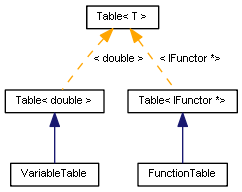Diagram of Variables and Functions table classes

A storage class is defined for storing all functors.

```  class AbstractFuncStorage
{
protected:
IFunctor *funcs[];
int total;

public:
~AbstractFuncStorage()
{
for(int i = 0; i < total; i++)
{
CLEAR(funcs[i]);
}
}
{
ArrayResize(funcs, total + 1);
funcs[total++] = f;
}
void fill(FunctionTable &table)
{
}
};

```

The 'fill' method fills the table with standard functions form the storage (the funcs array). To enable automatic passing of all created functors to the storage, create its static instance inside the base class of the AbstractFunc function and fill it with 'this' references from the constructor.

```  class AbstractFunc: public IFunctor
{
private:
const string _name;
const int _arity;
static AbstractFuncStorage storage;

public:
AbstractFunc(const string n, const int a): _name(n), _arity(a)
{
}
string name(void) const override
{
return _name;
}
int arity(void) const override
{
return _arity;
}
static void fill(FunctionTable &table)
{
storage.fill(table);
}
};

static AbstractFuncStorage AbstractFunc::storage;

```

Of course, the constructor receives input parameters enabling it to identify the name and arity of the function.

An intermediate template class FuncN has been added for declaring functions with special arity. Arity in this class is set by the size of the passed type (as for now function arity does not exceed 3 and there are no zero-size types, so we use a notation sizeof(T) % 4 — and thus size 4 produces arity 0).

```  template<typename T>
class FuncN: public AbstractFunc
{
public:
FuncN(const string n): AbstractFunc(n, sizeof(T) % 4) {}
};

```

Types with sizes from 0 to 3 are generated using macros.

```  struct arity0 { char x; };

#define _ARITY(N)   struct arity##N { char x[N]; };

_ARITY(1);
_ARITY(2);
_ARITY(3);

```

We also need lists of arguments to automate the generation of function descriptions.

```  #define PARAMS0
#define PARAMS1 params
#define PARAMS2 params,params
#define PARAMS3 params,params,params

```

Now, we can define a macro for a functor, based on the FuncN<T> class.

```  #define FUNCTOR(CLAZZ,NAME,ARITY) \
class Func_##CLAZZ: public FuncN<arity##ARITY> \
{ \
public: \
Func_##CLAZZ(): FuncN(NAME) {} \
double execute(const double &params[]) override \
{ \
return CLAZZ(PARAMS##ARITY); \
} \
}; \
Func_##CLAZZ __##CLAZZ;

```

Finally, here is a list of supported functions with names and number of arguments.

```  FUNCTOR(fabs, "abs", 1);
FUNCTOR(acos, "acos", 1);
FUNCTOR(acosh, "acosh", 1);
FUNCTOR(asin, "asin", 1);
FUNCTOR(asinh, "asinh", 1);
FUNCTOR(atan, "atan", 1);
FUNCTOR(atanh, "atanh", 1);
FUNCTOR(ceil, "ceil", 1);
FUNCTOR(cos, "cos", 1);
FUNCTOR(cosh, "cosh", 1);
FUNCTOR(exp, "exp", 1);
FUNCTOR(floor, "floor", 1);
FUNCTOR(log, "log", 1);
FUNCTOR(log10, "log10", 1);
FUNCTOR(fmax, "max", 2);
FUNCTOR(fmin, "min", 2);
FUNCTOR(fmod, "mod", 2);
FUNCTOR(pow, "pow", 2);
FUNCTOR(rand, "rand", 0);
FUNCTOR(round, "round", 1);
FUNCTOR(sin, "sin", 1);
FUNCTOR(sinh, "sinh", 1);
FUNCTOR(sqrt, "sqrt", 1);
FUNCTOR(tan, "tan", 1);
FUNCTOR(tanh, "tanh", 1);

```

The functor class diagram in a generalized form looks like this: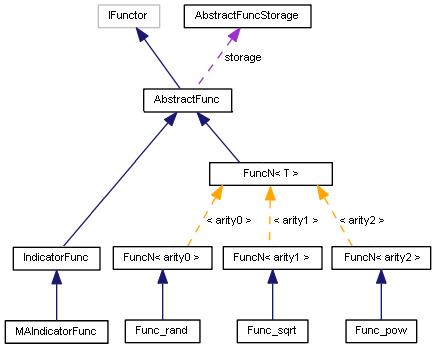Functor class diagram

The diagram does not show all functions, but only one function of each arity. It also has some functions which will be considered later.

And thus, everything is ready to use two recursive descent parsers. One of them calculates expressions in the interpretation mode. The other one calculates expressions using syntax trees.

### Evaluating expressions on the fly (ExpressionEvaluator)

The expression evaluation by the interpreter is as follows: we create an ExpressionEvaluator instance, pass variables to it if necessary, and call the 'evaluate' method with a string containing the required expression.

```  ExpressionEvaluator ee("a=-10");
double result = ee.evaluate("1 + sqrt(a)"); // -nan(ind)
bool success = ee.success();                // true

```

Using the 'success' method, we can check if the expression is syntactically correct. However, this does not guarantee that their will be no errors during calculations. In the above example, an attempt to extract root of a negative variable will return NaN. Therefore, it is recommended to check the result using the MathIsValidNumber function.

After developing other parsers, we will write tests with a more detailed description of the process.

### "Compiling" expressions into a syntax tree and evaluating the tree (ExpressionCompiler)

Evaluation of an expression by building a syntax tree is performed as follows: we create an instance of ExpressionCompiler, pass initial variables to it if necessary, and call the 'evaluate' method with a string containing the required expression. As a result, we receive a reference to the Promise object, for which we need to call 'resolve' in order to evaluate the expression and to get a number. This looks more cumbersome, but it works much faster when you need to perform multiple calculations for different values of variables.

```  double a = {...}, b = {...}, c = {...};

VariableTable vt;
ExpressionCompiler с(vt);
const string expr = "(a + b) * sqrt(c)";
Promise *p = c.evaluate(expr);

for(int i = 0; i < 10; i++)
{
vt.set("a", a[i]);
vt.set("b", b[i]);
vt.set("c", c[i]);
Print(p.resolve());
}

```

An empty table of variable is first created here. The changing values for variables a, b, c are written to this table in a loop. The adhocAllocation method which is used here, sets a flag instructing the parser to accept and reserve any variable names in the table, at the parsing and tree generation stage. Any such implicit variable is set to nan, so the caller must set them to real values before calculating the "promise".

If we do not call vt.adhocAllocation(true) before c.evaluate in the above example, all variables encountered in the expression will generate errors, because it is assumed by default that the variables must be described in advance but the table is empty. You can check for errors in your code by calling c.success() after c.evaluate(). Errors are also logged.

Similar to the interpreter, the 'evaluate' method will return some result anyway. So, if the variables are not known at the parsing stage, nodes with the nan value will be created for them in the tree. Computing on such a tree is useless since this will also return nan. But the presence of a tree allows understanding what the problem is. The Promise class has a helper method for printing the tree — print.

### Conclusion

In this article, we considered the basics of parsing mathematical expressions. We have also created two ready-to-work MQL parsers. A small test script is attached below, allowing you to start using the technology in your programs. We will continue to explore other parser types in the second part: we will compare their performance and give examples of how to use them for solving trader tasks.

Translated from Russian by MetaQuotes Software Corp.
Original article: https://www.mql5.com/ru/articles/8027

Attached files |
parsers1.zip (14.43 KB)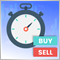Quick Manual Trading Toolkit: Basic Functionality

Today, many traders switch to automated trading systems which can require additional setup or can be fully automated and ready to use. However, there is a considerable part of traders who prefer trading manually, in the old fashioned way. In this article, we will create toolkit for quick manual trading, using hotkeys, and for performing typical trading actions in one click.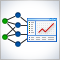Practical application of neural networks in trading. It's time to practice

The article provides a description and instructions for the practical use of neural network modules on the Matlab platform. It also covers the main aspects of creation of a trading system using the neural network module. In order to be able to introduce the complex within one article, I had to modify it so as to combine several neural network module functions in one program.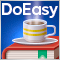Timeseries in DoEasy library (part 45): Multi-period indicator buffers

In this article, I will start the improvement of the indicator buffer objects and collection class for working in multi-period and multi-symbol modes. I am going to consider the operation of buffer objects for receiving and displaying data from any timeframe on the current symbol chart.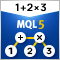Calculating mathematical expressions (Part 2). Pratt and shunting yard parsers

In this article, we consider the principles of mathematical expression parsing and evaluation using parsers based on operator precedence. We will implement Pratt and shunting-yard parser, byte-code generation and calculations by this code, as well as view how to use indicators as functions in expressions and how to set up trading signals in Expert Advisors based on these indicators.